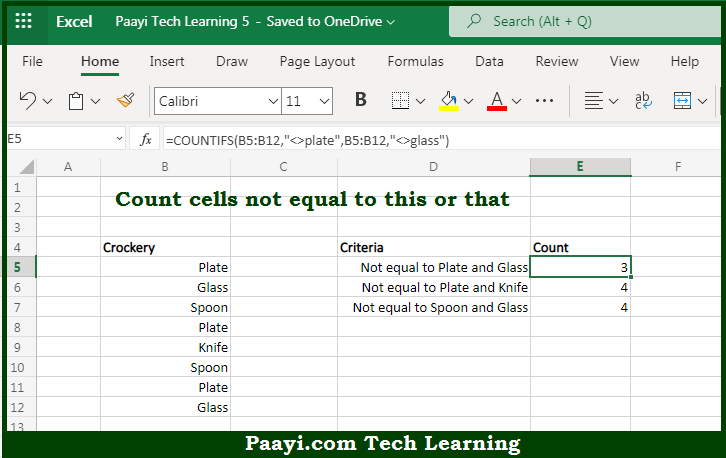# Learn How to Count Cells Not Equal x or y in Microsoft Excel

Written by | 0 Comments | 406 Views

In this article, you will learn how to COUNT various things in Microsoft Excel using a single or combination of functions and its purpose in Microsoft Excel. You will also get to know how to count cells not equal to x or y and see the generic formula.

Count Cells Not Equal x or y in Microsoft Excel

The main purpose of this formula is to count cells not equal to any particular thing. Here we will learn how to count cells not equal this and that in Microsoft Excel.  That implies, with the help of a formula based on the COUNTIFS function with multiple criteria you can able to count the cell snot equal to this or that. In the example shown below "rng" is the range used. So, with the help of this formula, you can able to count cells not equal to any particular thing.

General Formula to COUNT Cells Not Equal To x or y

=COUNTIFS(rng,"<>x",rng,"<>y")

The Explanation for the COUNT Cells Not Equal To x or ySo we know that Microsoft Excel COUNTIF function you can able to count cells not equal to any particular thing. Here we will learn how to count cells not equal this and that in Microsoft Excel. As we know that, The COUNTIFS function is used to count the number of cells in a range that meets one or more supplied criteria. Every condition must pass in order for a cell to be counted. It should be noted that criteria are supplied with range/criteria pairs, and can also use logical operators. So, with the help of the COUNT formula, you can able to count cells not equal to x or y.